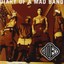MathematicsOpenStudy (anonymous):

can anyone help me on this problem? "Mary's age is twice Fred's age and 1/3 of Sally's age. If the sum of their ages is 99 how old are they?OpenStudy (shadowfiend):

Let's call Mary's age $$m$$, Fred's age $$f$$, and Sally's age $$s$$. Then: \begin{align} m &= 2f\\ m &= \frac{s}{3}\\ m + s + f &= 99 \end{align} We can solve this system of equations first by solving for $$s$$ in terms of $$m$$: $s = 3m$ We already have $$m$$ in terms of $$f$$, which means we have $$s$$ in terms of $$f$$ as well: $$s = 3m = 3(2f) = 6f$$. So we can plug into the last equation to solve for $$f$$: \begin{align} 2f + 6f + f &= 99\\ 9f &= 99\\ f &= 11 \end{align} Is this enough for you to find the other ages?OpenStudy (anonymous):

oh my goodness yes i think that helps SO much! THANK YOU!!!OpenStudy (shadowfiend):

Great!

Latest Questions5824234167: I hope you like this my voice cracked a little. Enjoy. Lovely Billie Eilish ft. K
19 minutes ago 2 Replies 0 Medalsbrianagatica14: What do you think of my spawn drawing? I would like to hear any advice and answer honstely.
32 minutes ago 7 Replies 4 MedalsRylee88: How do I write a fraction as a mixed number? ~Math
46 minutes ago 3 Replies 1 MedalJoe348: Is it rational to replace Columbus Day with Indigenous Peoples Day?
56 minutes ago 10 Replies 1 MedalJamesTDG: If f(x) = x^2 u2013 26 and g(x) = x u2013 4, what is the domain of (StartFraction f Over g EndFraction) (x)? Show your work! I changed the equation so I can
18 minutes ago 3 Replies 1 MedalSprinkles285: Answer the questions below. Use the rubric in the materials for help if needed. Your response should consist of three complete sentences in Spanish.
8 minutes ago 9 Replies 1 Medalkward64: ok i sung i forrogt the name of the song aready but i sung someones song and here it is https://voca.
37 minutes ago 26 Replies 1 MedalKyledaGreat: Your backpacker's guide contains a grid map of Paris, with each unit on the grid representing 0.
1 hour ago 4 Replies 0 MedalsTETSXPREME: help
1 hour ago 2 Replies 0 MedalsNoodlearms: The dashed triangle is the image of the solid triangle. The center of dilation is (6, 6).
1 hour ago 14 Replies 2 Medals